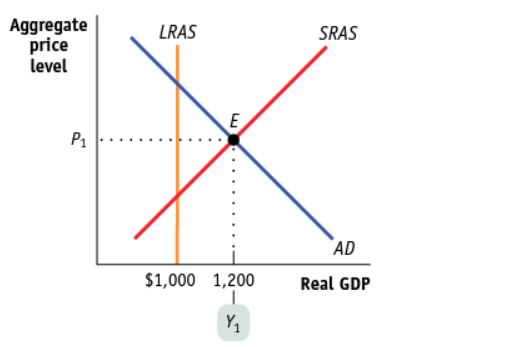Reading Guide: Section 4 Module 19
First Name *
Last Name *
Class Period *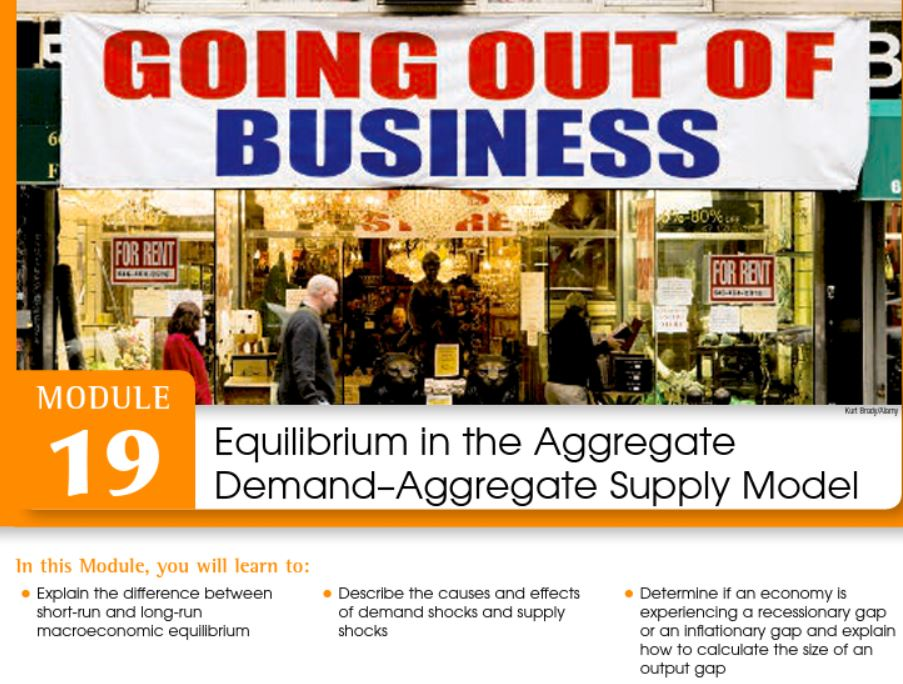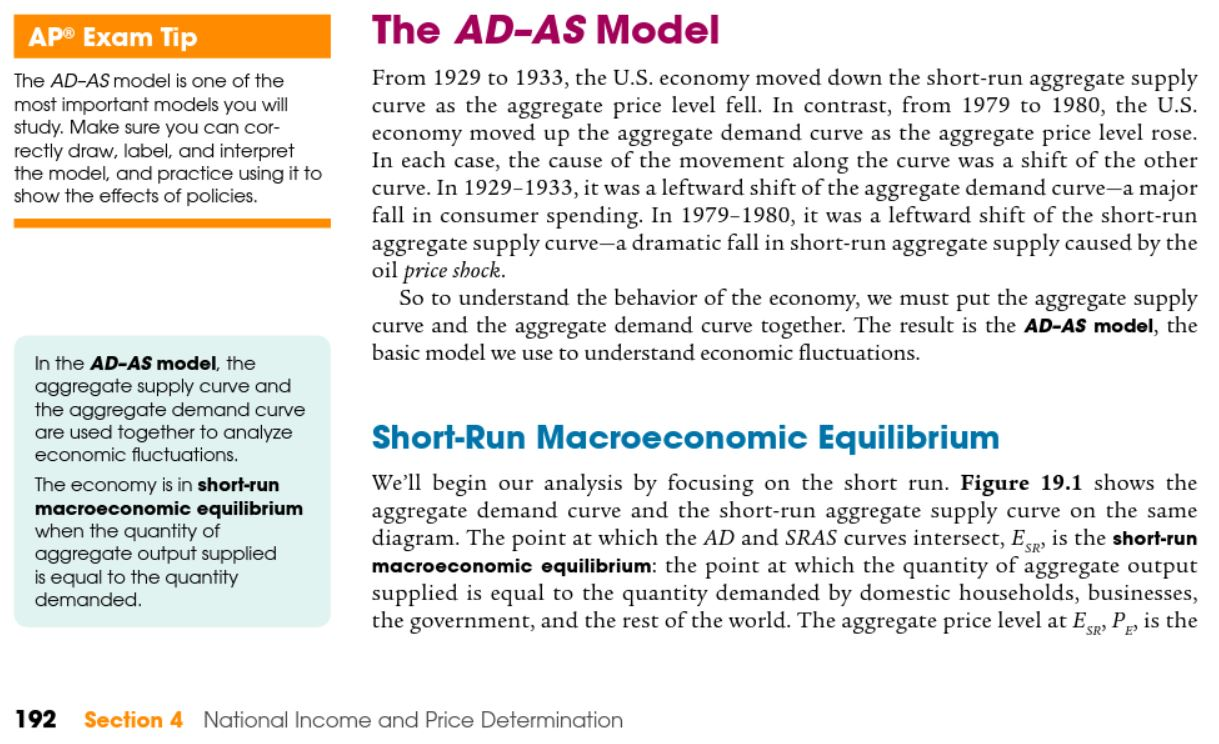1. Describe short-run macroeconomic equilibrium. *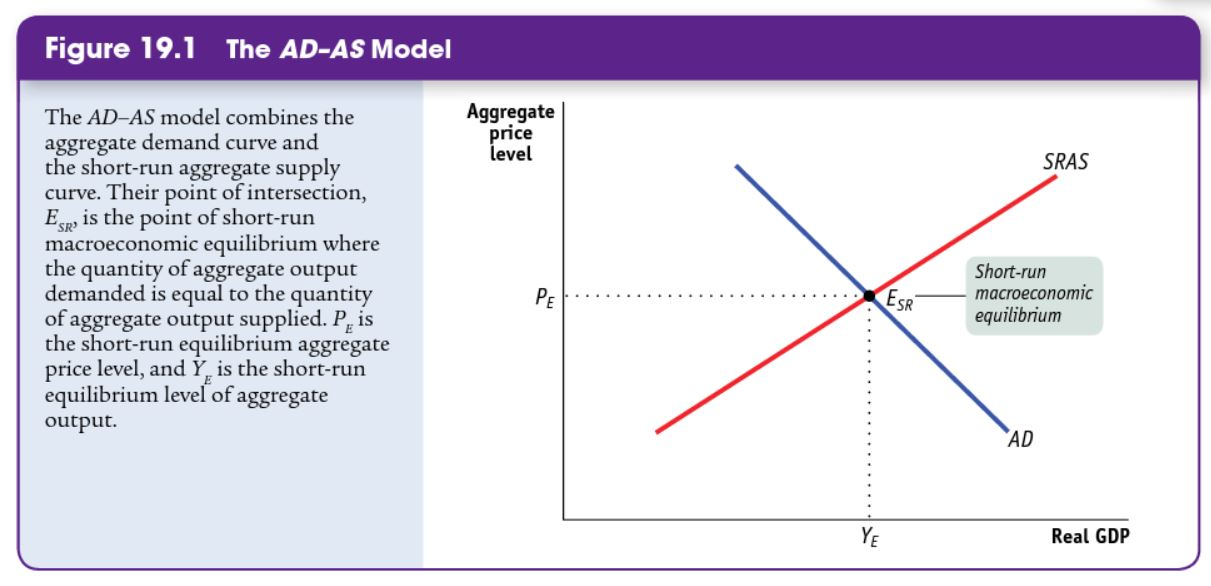2. At YE, what is in equilibrium? *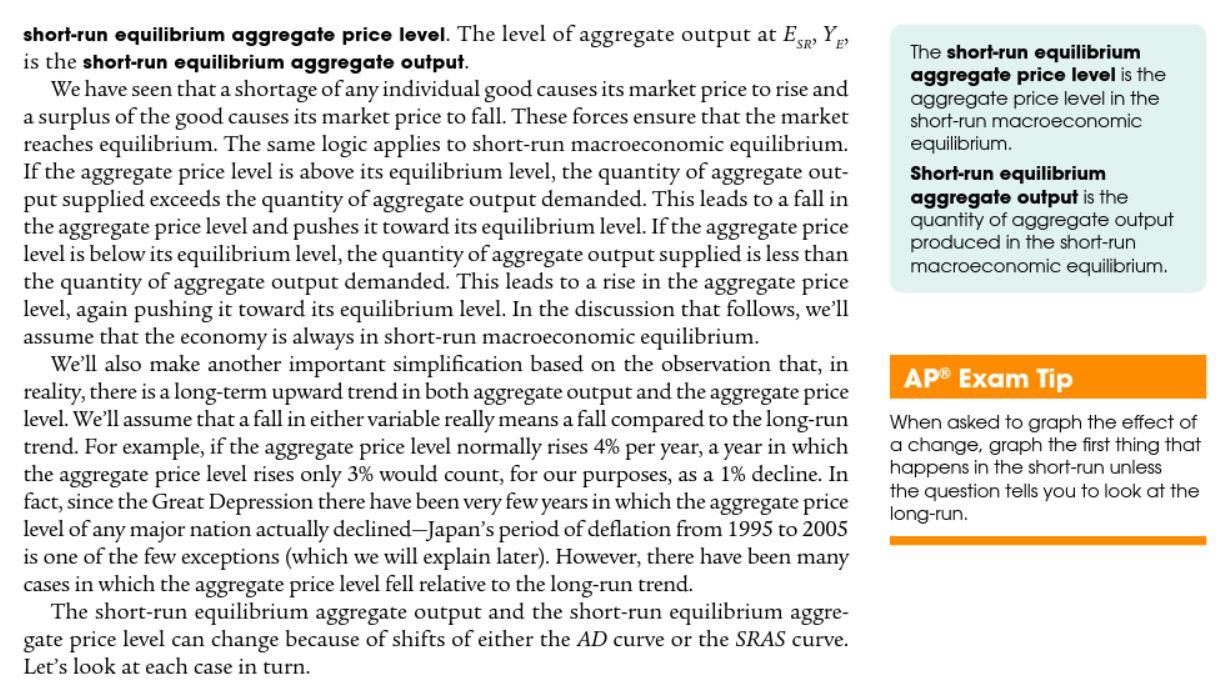3. Describe what can happen in the economy when the price level is above the equilibrium price level. *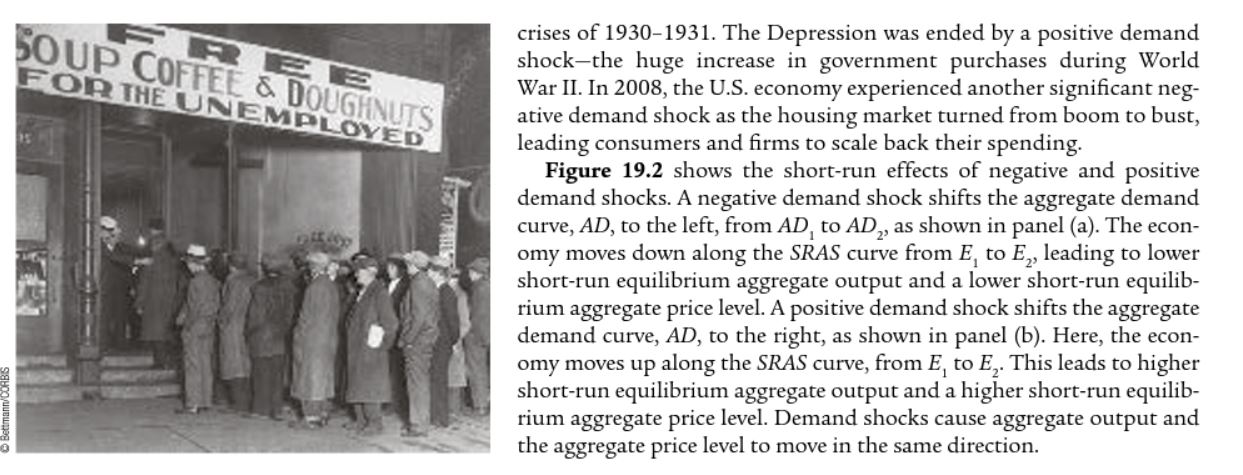4. Describe the impact of a demand shock on the economy. *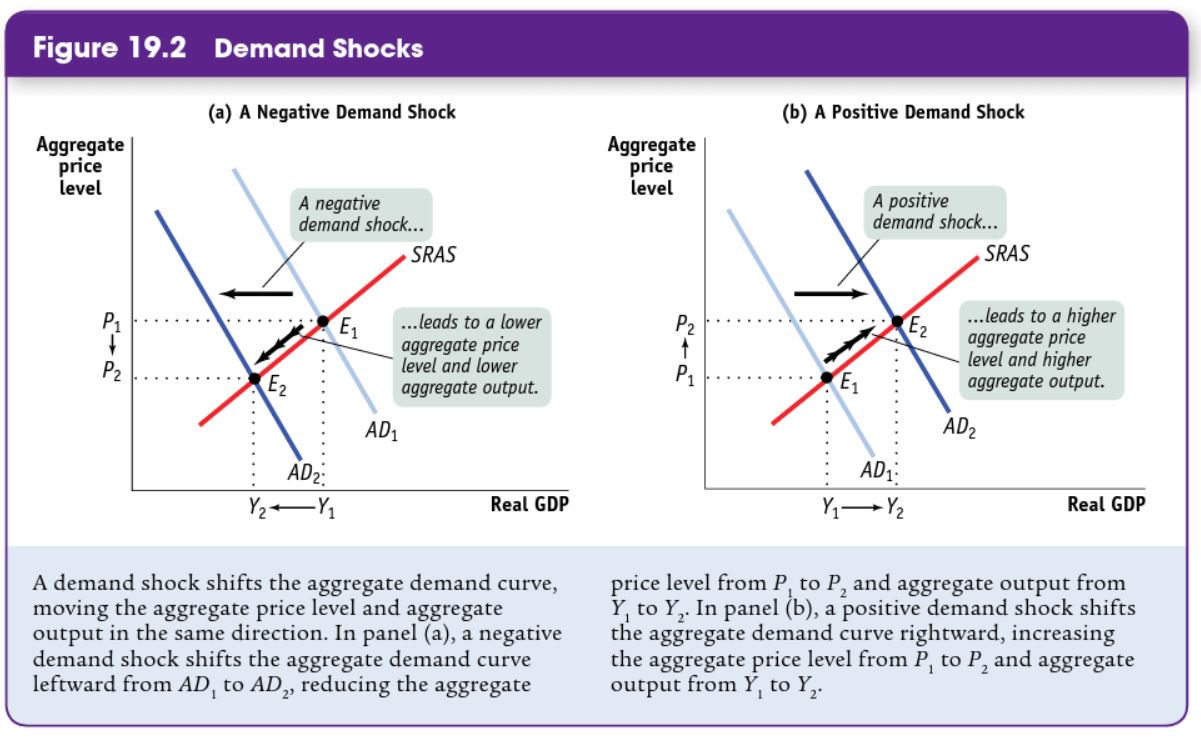5. Describe the difference between a negative demand shock and a positive demand shock. *6. Check all the true statements regarding the short-run aggregate supply curve if there is a negative supply shock: *
1 point
Required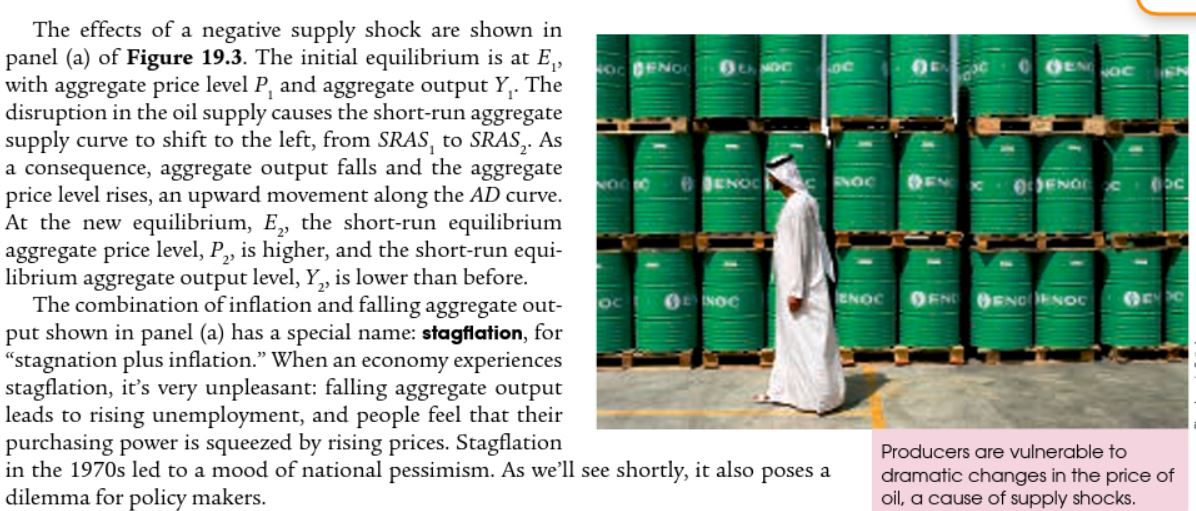7. Stagflation occurs when (check all that apply):
1 point8. Refer to the graphs above: What is the difference in RGDP and PL when SRAS shifts right versus left? *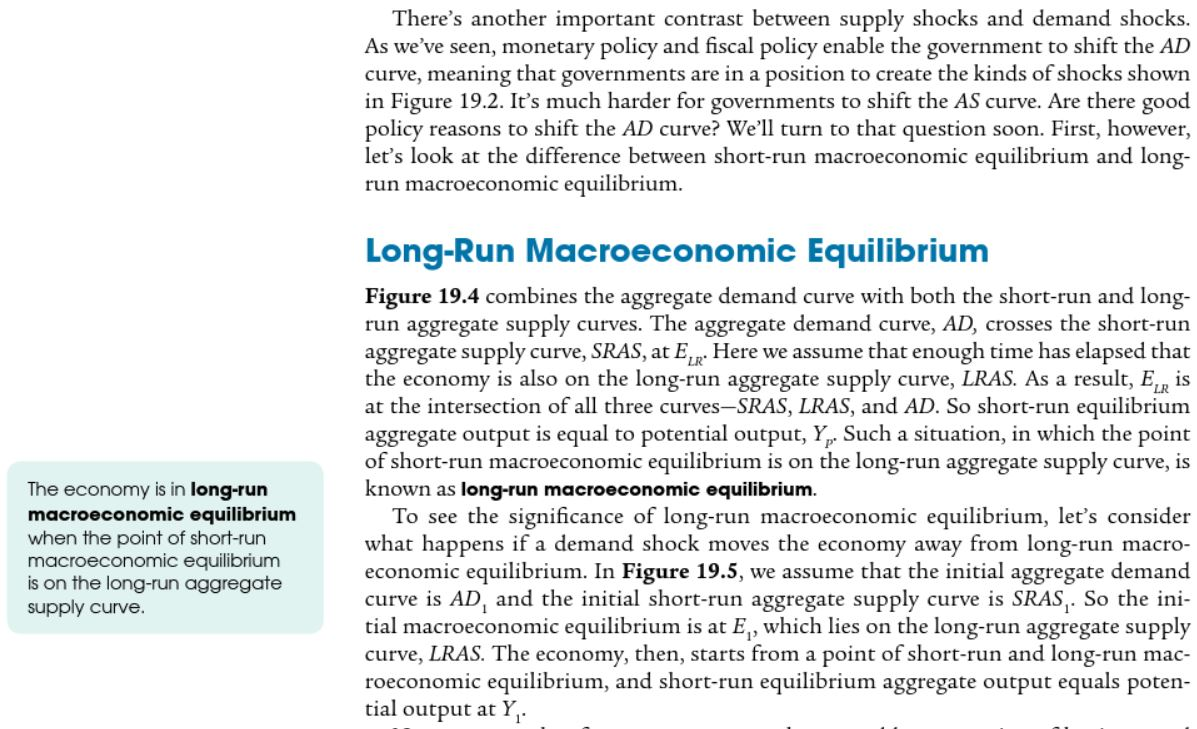9. Which of the following are TRUE if the economy is in long run equilibrium (choose all that apply)? *
Required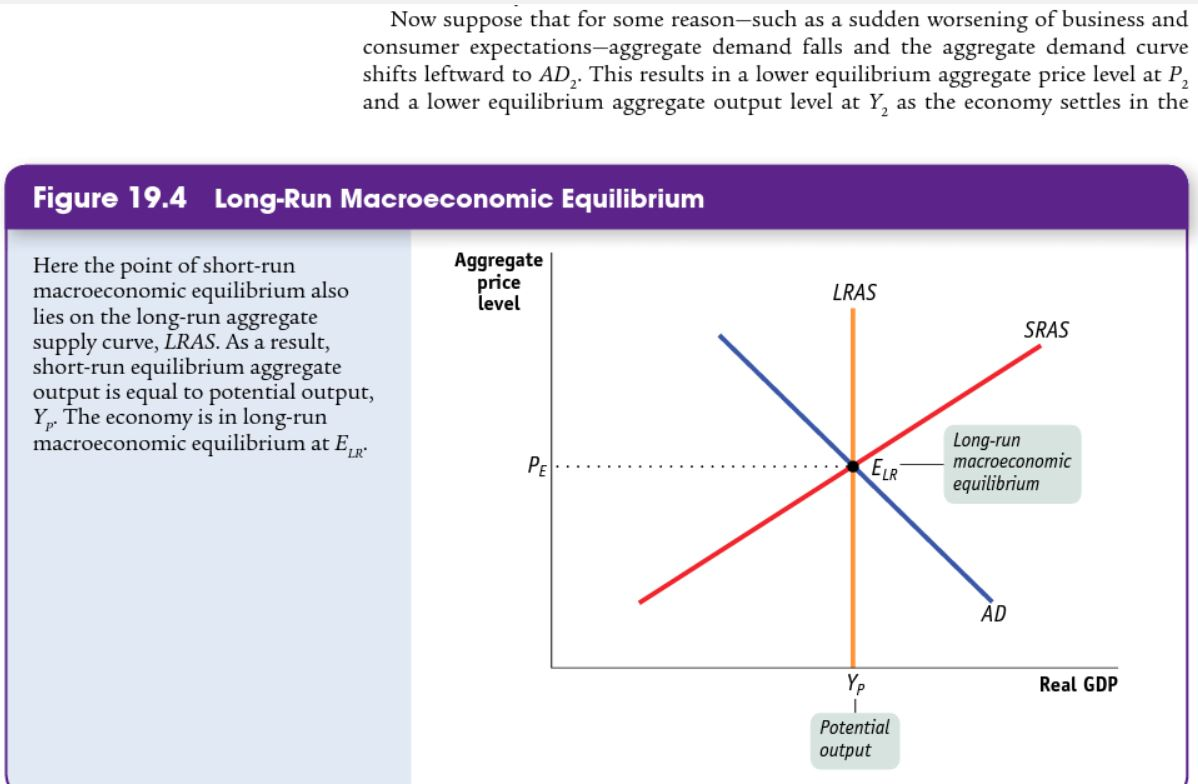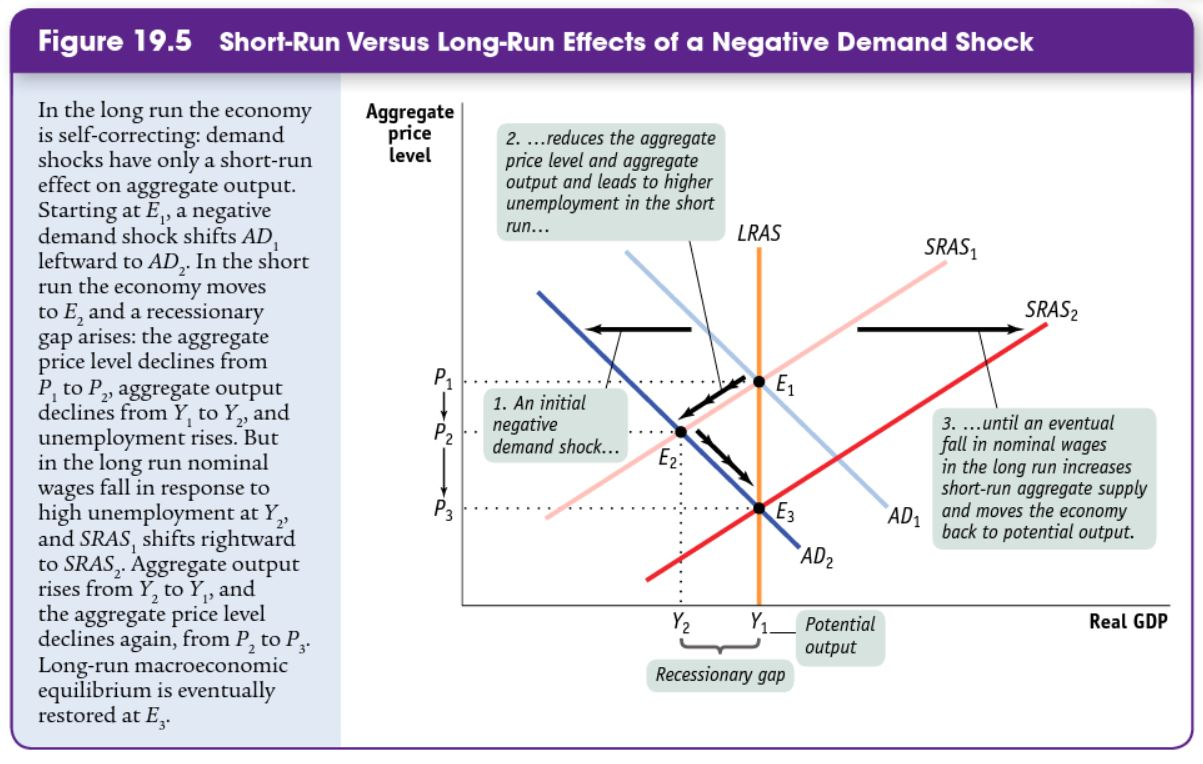**10. Explain how an economy recovers from a negative demand shock? More importantly, what happens to SRAS? *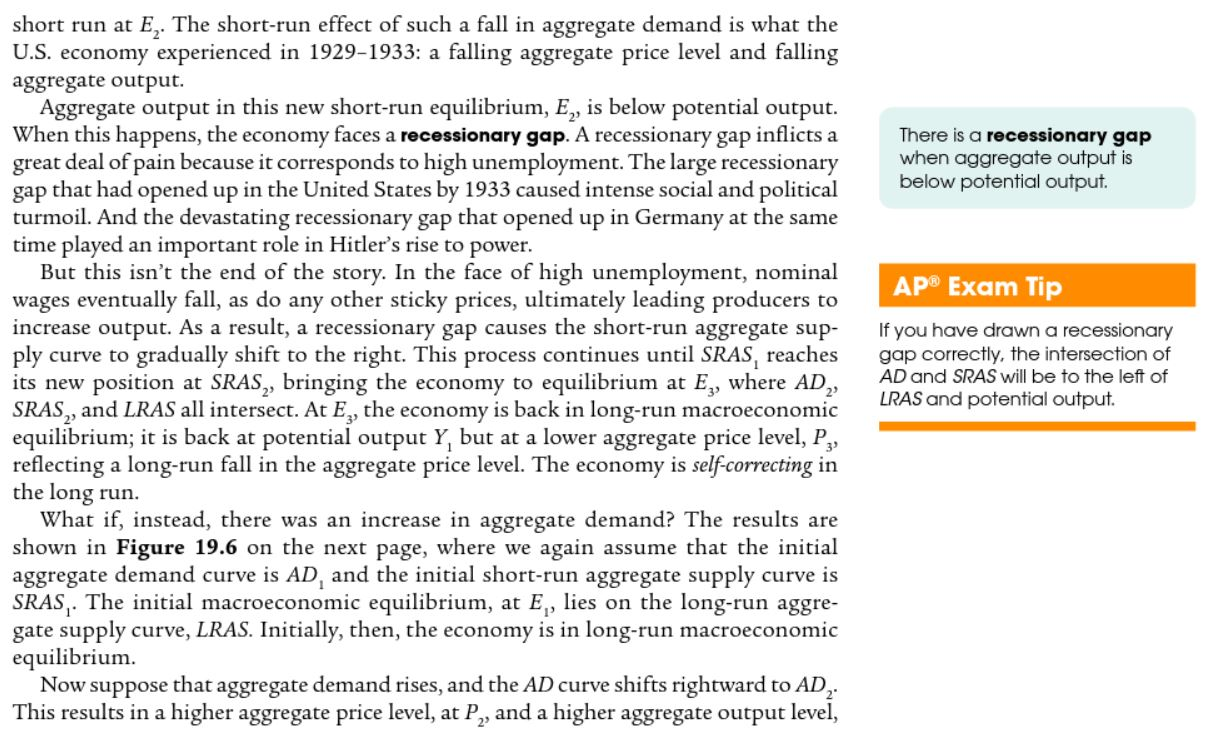11. Explain how an economy is "self-correcting" in the long run. READ WHAT YOU JUST SKIPPED TO ANSWER THIS! *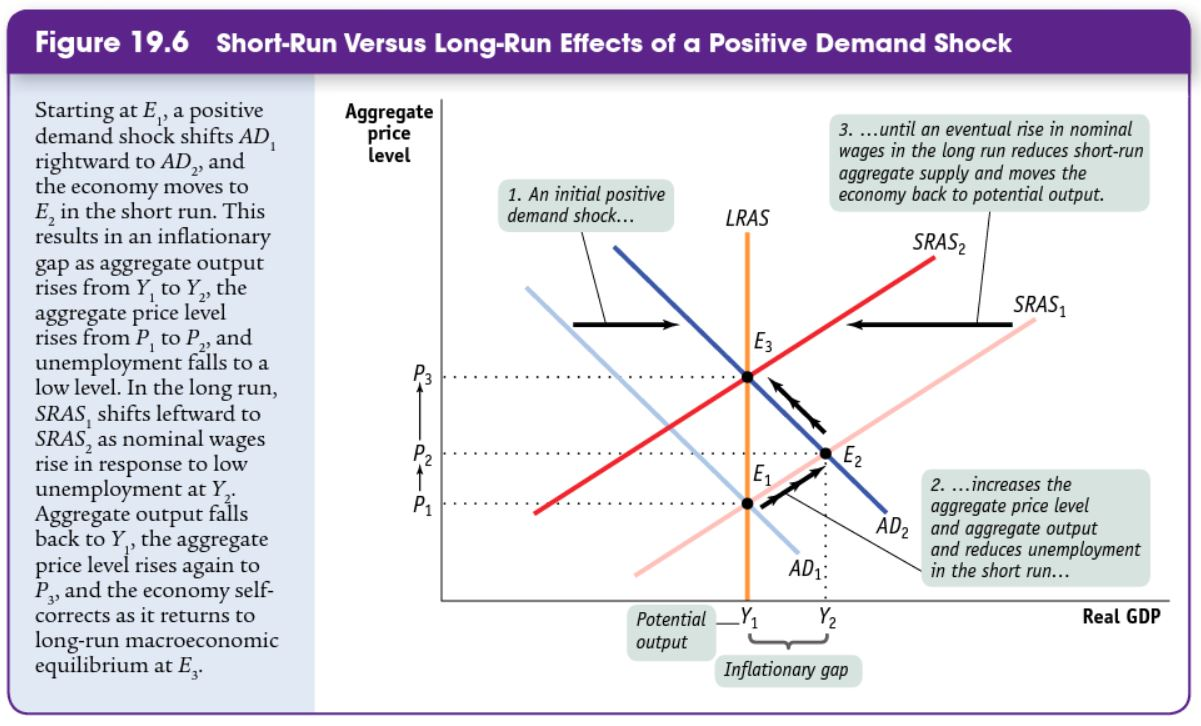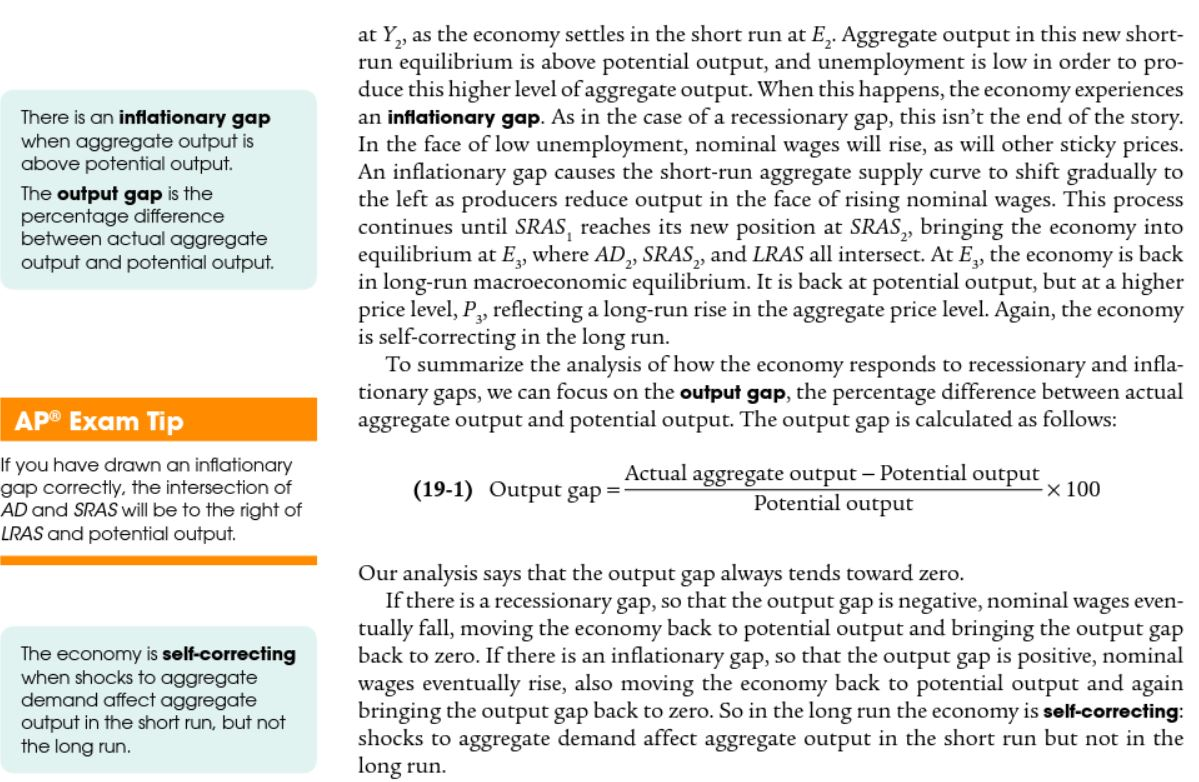12. Explain how an inflationary gap self corrects in the long-run. *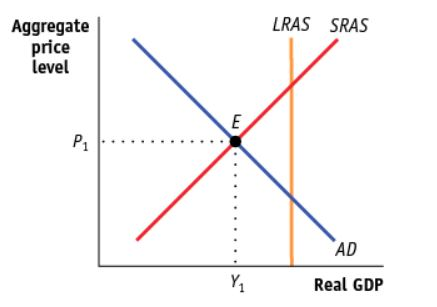13. The economy depicted above is in a(n): *
1 point
13.b. How will the economy above self correct in the long-run? *